The power (1)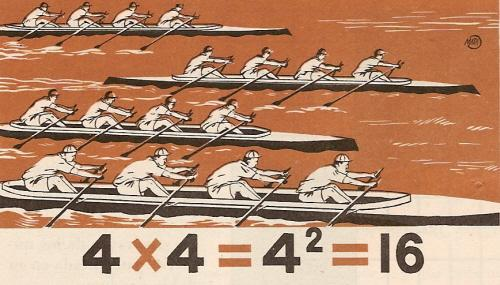1. Concept of the power

When we see the picture, we wonder: how many oarsmen participate in the regattas? There are 4 oarsmen in each of the four trawlers, then in total there are 4 x 4 = 16 oarsmen, This can also be written as: 42 and it is read "four to the power of two" .

The powers are products that are all equal factors.
Example: 5 x 5 = 52;  6 x 6 x 6 = 63;  7 x 7 x 7 x 7 = 74.

Do these problems on a paper and write the solution:

 1. The power 42 is... 2. The power 24 is... 3. The power 53 is... 4. The power 35 is... 5. The power 32 is... 6. The power 23 is...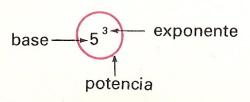2. - Elements of the power

In the power 53 we distinguish the base, which is 5 and the exponent, which is 3.
In this case 53 = 5 x 5 x 5. The base (5) is the number that is repeated in the multiplication. The exponent (3) is the number of times that we must multiply.

 1. In the power 73 the 7 is... 2. In the power 73 the 3 is... 3. In 84 the 8 is... 4. In 84 the 4 is... 5. In 52 the 2 is.... 6. In 52 the 5 is...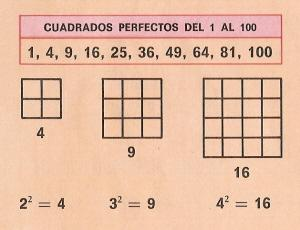3. - Perfect Squares

The powers with exponent 2 are called perfect squares.
In the picture, we see that the square of 2 (22) is 4, and the square of 3 (32) is 9, and the square of 4 (42) is 16. We can check it by counting the small squares.
When we studied the multiplication tables, we learned that 7 x 7 = 49. Now we can express it as: 72.

Exercises:

 1. The power 52 = 2. The power 82  = 3. The power 62 = 4. The power 92 = 5. The power 72 = 6. The power 102 =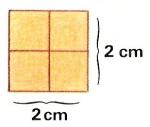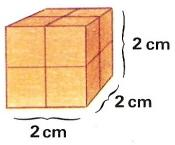4. - Squares and cubes

In the left picture, we see a square of 2 cm long and 2 cm width. In total there are 4 small squares of 1 cm side. 2 cm x 2 cm = 4 cm2

In the right picture, we see a cube that is 2 cm long, 2 cm wide and 2 cm high. In total, how many small cubes are there? Count them and you will see that there are 8 cubes. 2 x 2 x 2 = 23 = 8. 23 = 8.

Exercises:

 1. 52 = 2. 53 = 3. 63 = 4. 62 = 5. 72 = 6. 73 =

 | Educational applications | Mathematics | In Spanish  | To print

®Arturo Ramo García.-Record of intellectual property of Teruel (Spain) No 141, of 29-IX-1999
Plaza Playa de Aro, 3, 1ş DO 44002-TERUEL